Home > GC > Chapter 6 > Lesson 6.2.5 > Problem6-85

6-85.
1. For each shape below, use the geometric relationships to solve for the given variable(s). Show all work. Name the geometric relationships you used. Homework Help ✎

1.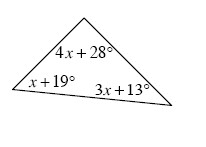2.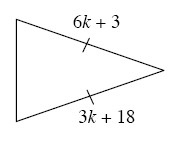3.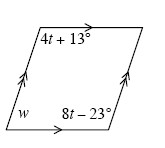4.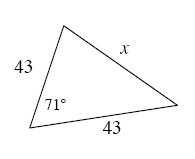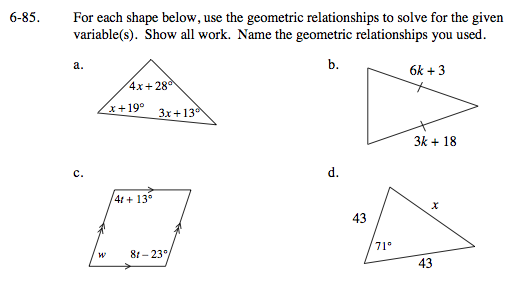What is the sum of the angles within a triangle?

x = 15°, Triangle Angle Sum

What do the tick marks on the sides of the triangles mean?

k = 5, Isosceles triangle

Extend the parallel sides.

Parallel lines have same side interior supplementary angles.

w + (4t + 13) = 180°
w + (8t − 23) = 180°

By the Equal Values Method: w + (8t − 23) = w + (4t + 13)

w + 8(9) − 23 = 180
w + 72 − 23 = 180
w + 49 = 180
w = 131°

4t = 36
t = 9°

Parallel lines

Use the Law of Cosines to solve for the unknown side.